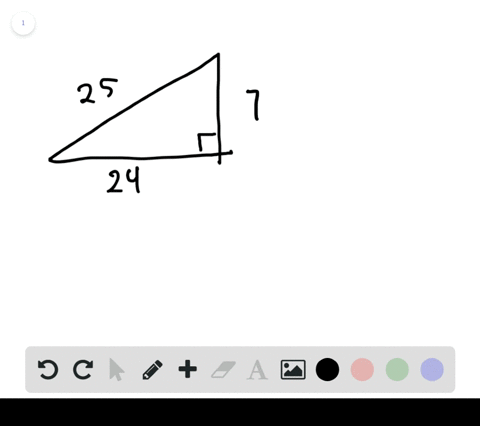🎉 The Study-to-Win Winning Ticket number has been announced! Go to your Tickets dashboard to see if you won! 🎉View Winning Ticket## Discussion

You must be signed in to discuss.

## Video Transcript

question two asks us to explain how someone would go about from converting a degree measure to a radiant. Now there's a simple formula that we can use to do this, and it's essentially multiplying our degree value by a conversion factor. And that conversion factor is to pie over 360 degrees. Now, why is this the conversion factor? Well, think about the unit circle on our unit circle. It's a circle, and how many degrees is a circle? Have 360. Okay? And if we look at the unit circle and think about it in terms of radiance, it has two pi radiance. So essentially these two things 360 degrees and two pi r equivalent to each other. And when they're put over each other in a fraction, when you multiply a degree measure by that you're essentially multiplying by one and converting it to radiance. So the simple formula to convert a degree measure into radiance is to multiply that degree by two pi over 360 degrees, and that's going to equal a radiant measure